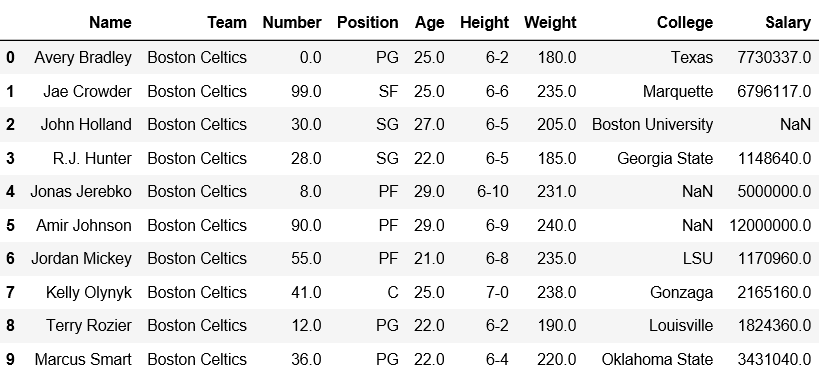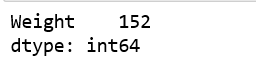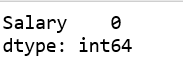# Get the index of minimum value in DataFrame column

Pandas DataFrame is two-dimensional size-mutable, potentially heterogeneous tabular data structure with labeled axes (rows and columns).

Let’s see how can we get the index of minimum value in DataFrame column.

Observe this dataset first. We’ll use ‘Weight’ and ‘Salary’ columns of this data in order to get the index of minimum values from a particular column in Pandas DataFrame.

 `# importing pandas module  ` `import` `pandas as pd  ` `   `  `# making data frame  ` `df ``=` `pd.read_csv(``"https://media.geeksforgeeks.org/wp-content/uploads/nba.csv"``)  ` ` `  `df.head(``10``) `Code #1: Check the index at which minimum weight value is present.

 `# importing pandas module  ` `import` `pandas as pd  ` `   `  `# making data frame  ` `df ``=` `pd.read_csv(``"nba.csv"``) ` ` `  `# Returns index of minimum weight ` `df[[``'Weight'``]].idxmin() `

Output:We can verify whether the minimum value is present in index or not.

 `  `  ` `  `# importing pandas module  ` `import` `pandas as pd  ` `   `  `# making data frame  ` `df ``=` `pd.read_csv(``"nba.csv"``) ` ` `  `# from index 140 to 154 ` `df.iloc[``140``:``155``] `

Output:Code #2: Let’s insert a new row at index 0, having minimum salary and then print the minimum salary.

 `# importing pandas module  ` `import` `pandas as pd  ` `    `  `# making data frame  ` `df ``=` `pd.read_csv(``"nba.csv"``) ` `  `  `new_row ``=` `pd.DataFrame({``'Name'``:``'Geeks'``, ``'Team'``:``'Boston'``, ``'Number'``:``3``, ` `                        ``'Position'``:``'PG'``, ``'Age'``:``33``, ``'Height'``:``'6-2'``, ` `                        ``'Weight'``:``189``, ``'College'``:``'MIT'``, ``'Salary'``:``99``} ` `                         ``, index``=``[``0``]) ` `  `  `df ``=` `pd.concat([new_row, df]).reset_index(drop``=``True``) ` `df.head(``5``) `

Output:Now, let’s check if the minimum salary is present at index 0 or not.

 `# Returns index of minimum salary ` `df[[``'Salary'``]].idxmin() `

Output:Whether you're preparing for your first job interview or aiming to upskill in this ever-evolving tech landscape, GeeksforGeeks Courses are your key to success. We provide top-quality content at affordable prices, all geared towards accelerating your growth in a time-bound manner. Join the millions we've already empowered, and we're here to do the same for you. Don't miss out - check it out now!

Previous
Next